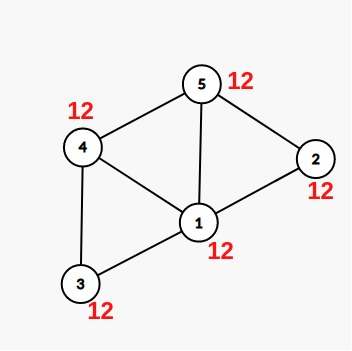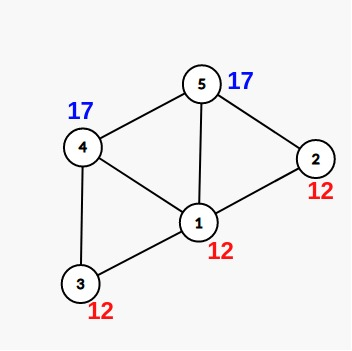# Practice on Toph

Participate in exhilarating programming contests, solve unique algorithm and data structure challenges and be a part of an awesome community.

# Tic Toc Toe

By asifthegreat · Limits 1s, 512 MB

We all know what Tic-Toc-Toe works. But in the anime world, people aren’t really interested in normal Tic-Toc-Toe. Their game is kinda different. Let’s see how to play it.

Firstly, you’re given an undirected graph witn $N$ nodes and $M$ edges. Every node has a cost. Initially all of them are 0. Now the game holder can update certain things and he will ask you some queries. So there are 2 types of operations in that game.

1. Update: $~$ Given node $U$ and a number $val$. You will start your journey at $U$ and you can move to a node $V$ if and only if $U \leq V$. So you can not pass through a node if it’s less than $U$. Let’s suppose we have a set $S$, which holds the nodes we can travel from $U$. You have to add $val$ to all the nodes in $S$.

2. Query: $~$ Given $U$ and you have to print the cost of the node $U$.

## Input

The first line will contain 2 numbers, $N$ ($2 \leq N \leq 10^5$) and $M$ ($2 \leq M \leq 10^5$), the number of nodes and the number of edges respectively.

Each of the next $M$ lines will contain 2 numbers $U, V$ ($1 \leq U, V \leq 10^5$) which means there is an edge between node $U$ and $V$.

The next line will contain a number $Q$ ($1 \leq Q \leq 10^5$), the number of operations.

Each of the next $Q$ lines can contain 2 types of lines:

• 1 $U$ val (update operation)

• 2 $U$ (Query operation)

NOTE: See the sample to be more clear.

## Output

For every query (second operation), print the cost of the node $U$ in a new line.

NOTE: See the sample to be more clear.

## Sample

InputOutput
5 7
1 2
1 3
1 5
1 4
2 5
4 5
4 3
4
1 1 12
2 5
1 4 5
2 5

12
17


### Explanation of the sample:

Here is the tree after the first operation:Here is the tree after second operation:•Graph

### Statistics

0% Solution Ratio

### Submit